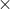In: Chemistry

# Calculate the pH of the solution that results from each of the following mixtures. 140.0 mL...

Calculate the pH of the solution that results from each of the following mixtures.

140.0 mL of 0.27 M  HF with 225.0 mL of 0.30 M  NaF

175.0 mL of 0.12 M C2H5NH2 with 265.0 mL of 0.20 M C2H5NH3Cl

## Solutions

##### Expert Solution

pH of the solution for an acid is calculated using Henderson-Hasselbach equation given by:

pH = pKa+log[acid]/[conjugate base]

pH of the solution for a base is calculated using Henderson-Hasselbach equation given by:

pOH = pKb+log[base]/[conjugate acid]

pH of the solution for the mixture 140.0 mL of 0.27M HF with 225.0 mL of 0.30M NaF is calculated as follows:

Number of moles of HF = 0.140L0.27M = 0.038

Number of moles of F- = 0.225L0.30M = 0.068

Total volume of the mixture = 0.140L+0.225L = 0.365L

[HF] = 0.0380.365 = 0.104M

[F-] = 0.0680.365 = 0.186M

pKa for HF is 3.18

Therefore, pH = 3.18+log[F-][HF] = 3.18+log0.1860.104

pH = 3.43

pH of the solution for the mixture 175.0 mL of 0.12M C2H5NH2 with 265.0 mL of 0.20M C2H5NH3Cl is calculated as follows:

Number of moles of C2H5NH2 = 0.175L0.12M = 0.021

Number of moles of C2H5NH3Cl  = 0.265L0.20M = 0.053

Total volume of the mixture = 0.175L+0.265L = 0.440L

[C2H5NH2] = 0.0210.440 = 0.048M

[C2H5NH3Cl ] = 0.0530.440 = 0.120M

pKb for C2H5NH2 is 3.19

Therefore, pOH = 3.19+log[C2H5NH3+ ][C2H5NH2] = 3.19+log0.1200.048

pOH = 3.59

Therefore, pH = 14-pOH = 14-3.59

pH = 10.41

## Related Solutions

##### Calculate the pH of the solution that results from each of the following mixtures. 1)140.0 mL...
Calculate the pH of the solution that results from each of the following mixtures. 1)140.0 mL of 0.24 M HF with 230.0 mL of 0.31 M NaF 2)185.0 mL of 0.12 M C2H5NH2 with 265.0 mL of 0.21 M C2H5NH3Cl
##### Calculate the pH of the solution that results from each of the following mixtures. a) 140.0...
Calculate the pH of the solution that results from each of the following mixtures. a) 140.0 mL of 0.24 M HF with 220.0 mL of 0.30 M NaF b) 165.0 mL of 0.12 M C2H5NH2 with 285.0 mL of 0.22 M C2H5NH3Cl Express your answer using two decimal places.
##### Calculate the pH of the solution that results from each of the following mixtures. a) 50.0...
Calculate the pH of the solution that results from each of the following mixtures. a) 50.0 mL of 0.17 molL−1 HCOOH (Ka=1.8×10−4) with 80.0 mL of 0.12 molL−1 HCOONa Express your answer using two decimal places. b) 135.0 mL of 0.13 molL−1 NH3 (Kb=1.76×10−5) with 240.0 mL of 0.13 molL−1 NH4Cl​
##### Calculate the pH of the solution that results from each of the following mixtures: a) 160.0...
Calculate the pH of the solution that results from each of the following mixtures: a) 160.0 mL of 0.24 M HF with 220.0 mL of 0.31 M NaF b) 185.0 mL of 0.12 M C2H5NH2 with 265.0 mL of 0.22 M C2H5NH3Cl
##### Calculate the pH of the solution that results from each of the following mixtures. 1) 50.0...
Calculate the pH of the solution that results from each of the following mixtures. 1) 50.0 mL of 0.16 M HCHO2 with 80.0 mL of 0.11 M NaCHO2 2)115.0 mL of 0.13 M NH3 with 250.0 mL of 0.13 M NH4Cl
##### : Calculate the pH of a solution that results from 125 mL of 0.10M NH3 with...
: Calculate the pH of a solution that results from 125 mL of 0.10M NH3 with 250 mL of 0.10 M NH4Cl; Kb = 1.76 x 10-5 Calculate the pH when 0.010 mols of NaOH are added to 250.0 mL buffer containing 0.250 M acetic acid and 0.250 M sodium acetate. (pka = 4.74) A 50.0 mL sample of 0.200M NaOH is titrated with 0.200M nitric acid. Calculate the pH after 25.0 mL HNO3 has been added.
##### Calculate the pH of each of the following mixtures. a) 25.00 ml 0.2875 M HCl mixed...
Calculate the pH of each of the following mixtures. a) 25.00 ml 0.2875 M HCl mixed with 12.25 ml 0.3548 M KOH b) 25.00 ml 0.2875 M HCl mixed with 35.25 ml 0.3548 M KOH c) What is the pH of a solution containing 0.52 M HBr and 1.25 M CH3CO2H. d) 25.00 ml 0.2875 M HOCl (ka = 3.5 x 10-8) mixed with 12.25 ml 0.3548 M KOH e) 25.00 ml 0.2875 M HOCl (ka = 3.5 x 10-8)...
##### 78 A.) Calculate the pH of the solution that results from mixing 20.0 mL of 0.022...
78 A.) Calculate the pH of the solution that results from mixing 20.0 mL of 0.022 M HCN(aq) with 80.0 mL of 0.066 M NaCN(aq). pH= b. Calculate the pH of the solution that results from mixing 28.0 mL of 0.117 M HCN(aq) with 28.0 mL of 0.117 M NaCN(aq). C. Calculate the pH of the solution that results from mixing 66.0 mL of 0.057 MHCN(aq) with 34.0 mL of 0.034 M NaCN(aq).. The Ka value for HCN is 4.9×10^(−10)...
##### calculate the pH of a solution that results from the addition of the following amounts of...
calculate the pH of a solution that results from the addition of the following amounts of 0.1 M NaOH to 10mL of a 0.1 HCl solution. Find the pHs for the following volumes of 0.1M NaOH added: 1, 2, 4,8, 9.8, 10.1, 10.2, 10.4, 11, 14, 18, 20 (all in mL)
##### 1. Calculate the pH of a solution that results from mixing 15 mL of 0.13 M...
1. Calculate the pH of a solution that results from mixing 15 mL of 0.13 M HBrO(aq) with 11 mL of 0.1 M NaBrO(aq). The Ka value for HBrO is 2 x 10-9. 2. What is the buffer component ratio, (BrO-)/(HBrO) of a bromate buffer that has a pH of 7.91. Ka of HBrO is 2.3 x 10-9.